STAT 804: Lecture 19 Notes

Processes with Periodic Components

Some of the series we have looked at have had clear annual cycles, returning to high levels in the same month every year. In our analysis of such processes we have tried to model the mean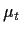as a periodic function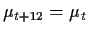. Sometimes we have fitted specific periodic functions to- writing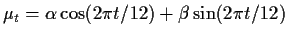.

Another process we studied, that of sunspot numbers, also seems to show a clearly periodic component, though now the frequency or period of the oscillation is not so obvious. In this section of the course we investigate the notion of decomposing a general stationary time series into simple periodic components. We will take these components to be cosines and sines. We will be focussing on problems in which the period is not prespecified, that is problems more like the sunspot data than the annual cycle examples.

For a statistician, the simplest description of what we will do is to say that we will examine the correlation between our series and sines and cosines of various periods. We will use these correlations in several ways:

• We will look for periods for which the correlation is very high to seek evidence of an underlying entirely periodic component with that particular period.

• We will study the behaviour of these correlations as a function of period (or frequency) for ARMA processes. The resulting plots can be used to identify specific ARMA models and to test the quality of an ARMA fit.

• We will study the effect of filtering on these correlations. Different filters can remove periodic components (and diminish the correlation between the filtered process and the sine or cosine of corresponding frequency).

• We can use this information about the effect of filtering to discuss the fitting of models to pairs of series in which we assume that a series Y is a filtered version of a series X, both of which we have data from, and try to estimate the filter.

Periodic Functions

A periodic function f on the real line has the property that f(t+d)=f(t) for some d and all t. The smallest possible choice of d is the period of f. The frequency of f in cycles per time unit, is 1/d. The most famous periodic functions are the trigonometric functions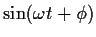and its relatives. This function has period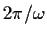and frequency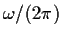cycles per time unit. Often, for trigonometric functions it is convenient to refer to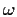as the frequency; the units now are radians per time point.

The achievement of Fourier was to recognize that essentially any function f with period 1 can be represented as a sum of functions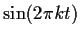or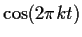. The tactic is to suppose that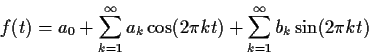To discover the values of the coefficients we make use of the orthogonality properties: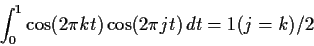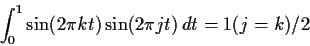and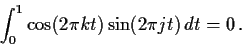Now multiply f(t) by sayand integrate from 0 to 1. Expanding the integral using the supposed expression of f as a sum gives us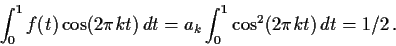Similarly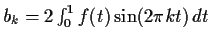.

Mathematically the fact that we can derive a formula for the coefficients is far from proving that the resulting sum actually represents f; the key missing piece of the proof is that any function whose Fourier coefficients are all 0 is essentially the 0 function.

Correlation between functions

The integrals in the previous section can be thought of as analogous to covariances and variances. For instance a Riemann sum for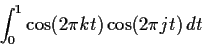is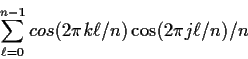which is an average product. In fact it is possible to show that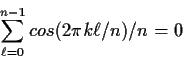So that the average product is just a sample'' covariance. It is also possible to evaluate the average product exactly to see that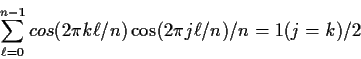exactly. When j=k this becomes a variance, equal to 1/2 so that the correlation is just the covariance times 2, which is 0 in any case when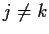.

Interpreting all the integrals above, then, as covariances we see that all the sines are uncorrelated with each other and with all the cosines and all the cosines are uncorrelated with each other.

Notice particularly that the sine with frequency j and the cosine with frequency j are uncorrelated. This has an important implication for looking for components at frequency j cycles per time unit in a time series: if we want a certain frequency we have to consider both the cosine and the sine at that frequency. An alternative summary of what we need is to consider the trigonometric identity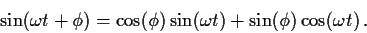When we look for a component with frequencywe will allow ourselves to adjust the number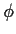, called the phase, in order to mazimize the correlation with our data. This is equivalent to adjusting the coefficients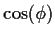and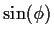to maximize a correlation with the right hand side of the trigonometric identity.

Complex Exponentials

Many of the identities in this subject are more easily derived using complex variables. In particular, the identity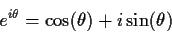where i2=-1 permits any series in sines and cosines to be rewritten in terms of exponentials. We can then often use tricks involving geometric sums to simplify our algebra.

For instance we can write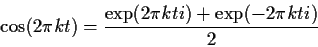and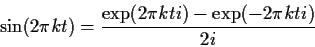These permit us to rewrite the expansion (1) in the form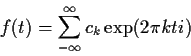where ck= (ak-ib)/2k for k>0, c0=a0 and ck=(ak+ibk)/2 for k<0. In fact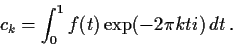Fourier transforms

For functions which are not periodic we can proceed by a further approximation Suppose f is defined on the real line and fix a large value of T. Define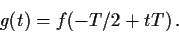Then g is defined on [0,1] and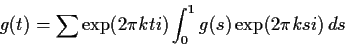according to (1) above. Re-express the conclusion in terms of f to get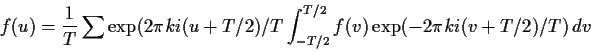which simplifies to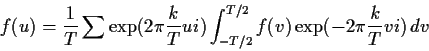You should recognize this sum as a Riemann sum for the integral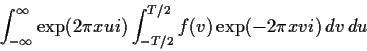which then converges as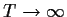to the expression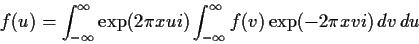The function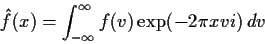is called the Fourier transform of f and we have derived a Fourier inversion formula. [WARNING: no proofs here! This integral will exist for, for example, f which are integrable over all the real line. ] This inversion formula expresses the function f as a linear combination of sines and cosines, though there are infinitely many frequencies involved.

Transforms of Stochastic Processes

We now seek to apply these ideas with the function f being our stochastic process X. We have several difficulties:

• X is not periodic.

• X is often only a discrete time function and in any case our data is inevitably discrete time.

• Even for continuous time X, the integral in the Fourier transform will typically not converge since X does not even go to 0 at plus or minus infinity.

The discrete nature of X leads us to the study of a discrete approximation to the integral: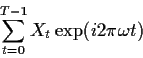This object has real part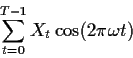and imaginary part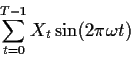so that apart from the means not being 0 we are studying the sample covariance with sines and cosines at frequency. We now study the statistical properties of these objects and then try to interpret them.

Suppose that X is a mean 0 stationary time series with autocovariance function C. We define the discrete Fourier transform of X as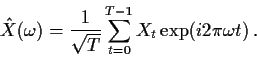Our choice to divide by the square root of T is motivated by the recognition that the sum of T terms typically has a standard deviation on the order of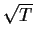leading us to expect that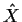will have a standard deviation which has a reasonable limit as.

We begin by computing moments of. Sinceis complex valued we have to think about what these moments should be. One way to think about this is to viewas a vector with two components, the real and imaginary parts. This would givea mean and a 2 by 2 variance covariance matrix. Also of interest however will be the expected modulus squared of, namely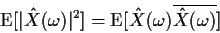where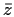is the complex conjugate of z. (If z=x+iy with x and y real then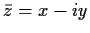.)

Since the Xs have mean 0 we see that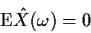(you should note that the expected value of a complex valued random variable is computed by finding the expected value of the real and imaginary parts). Then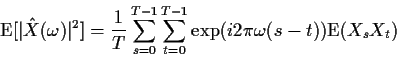The expected values are just C(s-t). We can gather together all the terms involving C(0), all those involving C(1) and so on to find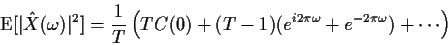which simplifies to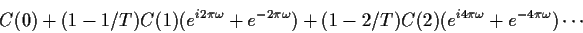Asthe coefficents of C(k) converges to 1 and we see (using C(k)=C(-k))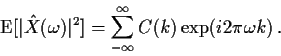The right hand side of this expression is defined to be the spectral density, or power spectrum, of X: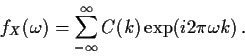There are a number of ways to look at spectral densities and the discrete Fourier transform:

• The discrete Fourier transform is actually a rerepresentation of the data because we can recover the data from the transform by an inverse transform: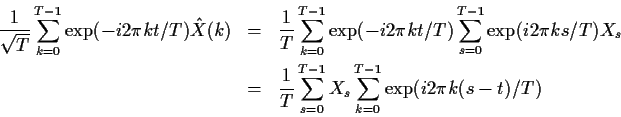For s=t the sum over k is simply T while for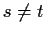the sum can be done as a geometric series and seen to be 0. Thus the inside sum just picks out the term s=t giving Xt as the inverse transform.

• Thus the DFT decomposes X into trigonometric functions of various frequencies with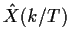being the weight on the component at frequency k/T. The spectral density is the limit of the variance of that weight or an approximation to the variance of the component of X at frequency k/T.

• The spectral density is a transform of the autocovariance function of X. In particular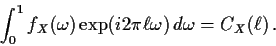• Since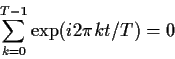for any integer t we see thatis, apart from a factor of sqrtT, a complex number whose real part is the sample covariance between X and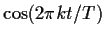and whose imaginary part is the sample covariance between X and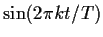. Consider computing the covariance between X and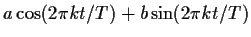with a and b chosen to maximize the covariance subject to a2+b2=1. The resulting coefficients are found by multiple regression of Xt on the cosine and sine. Using the fact that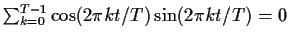we can check that the covariance is maximized by taking a and b proportional to the real and imaginary parts ofrespectively and that the covariance with this linear combination is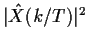. This calculation requires t to be a non-zero integer. In practice we usually apply these techniques to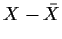.

• In view of the last calculation it is useful to examine the behaviour ofwhen X has a purely periodic component, say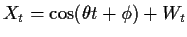where W is a stationary mean 0 series. We use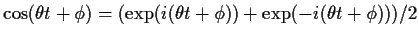to see that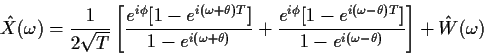The leading term converges to 0 unless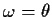or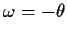. Restricting our attention to positive frequencies, whenwe get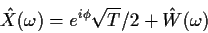If we movearound and look at the size ofwhen we hit a frequency at which there is a purely periodic component the modulus ofwill suddenly contain a component proportional to. This permits us to look for truly periodic components by looking for spikes or peaks in plots of the modulus squared of the DFT.

• We will see later that if two series Y and X are related by Y being a filtered version of X then the spectral densities have a very simple relation to one another in terms of some property of the filter. We can use this fact to actually estimate the filter itself when this is unknown.Richard Lockhart
1999-09-19